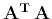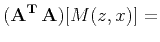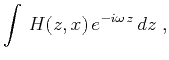Asymptotic pseudounitary stacking operatorsNext: Bibliography Up: Asymptotic pseudounitary stacking operators Previous: Conclusions

# Acknowledgments

I owe my familiarity with the asymptotic inversion theory to Sergey Goldin. A short discussion with Martin Tygel helped me better understand the true-amplitude migration concept.

I thank Jon Claerbout for helpful discussions and the sponsors of the Stanford Exploration Project for the financial support of this work. Comments from three anonymous reviewers helped to improve the paper.

# Least-squares Radon transform inversion

This appendix exemplifies the application of adjoint operators by reviewing the analytical least-squares inversion of the classic Radon transform (slant stack operator).

Forming the productfor this case leads to the double integral(88)

Applying Fourier transform with respect to, we can rewrite equation (A-1) in the frequency domain as(89)

where(90)(91)

The inner integral in equation (A-2) reduces to the-dimensional delta function:(92)

As follows from the properties of delta function,(93)

Inverting (A-6) for, we conclude that(94)

Substituting equation (A-7) into (13) produces the result precisely equivalent to Radon's inversion (4).Asymptotic pseudounitary stacking operatorsNext: Bibliography Up: Asymptotic pseudounitary stacking operators Previous: Conclusions

2013-03-03